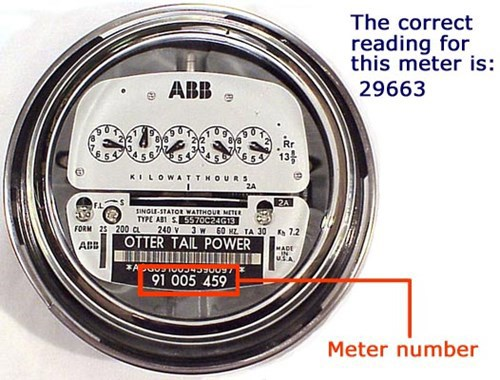### Digital meters

Some electric meters are digital. They look and read like the odometer on a car. Each meter is numbered. Note your meter number.

### Dial meters

Other electric meters have multiple dials, each with a pointer and the numerals 0 to 9. Below is an example of a five-dial electric meter. Notice that the pointers on the first, third, and fifth dials move clockwise. The second and fourth pointers move counter clockwise.Here's how to read a dial meter:

• Stand directly in front of the meter so that you clearly can see the location of each pointer.
• If the pointer is between two numbers, read the lower number.
• If the pointer is between 9 and 0, read 9.
• If the pointer appears to be exactly on a number, read the next lower number unless the pointer on the dial to your right has passed zero. You must read the dial on the far right independently.
• Each meter is numbered. Note your meter number.

Let's read this sample electric meter• Start on the far right . The pointer on the far right is directly on 5. Read it as 5.
• The next pointer has just passed 9 and is between 9 and 0. Read it as 9.
• The next pointer has passed 8 and is between 8 and 9. Read the smaller number: 8.
• The pointer on the next dial is directly on the 4 but, because the dial to the right has not passed zero, read this dial as 3.
• The pointer on the far left dial is between 8 and 9. Read the smaller number: 8.Current reading 83895 Minus last reading - 83770 Electricity use = 125 The difference of 125 kilowatt-hours is the amount of electricity used since the last reading.

Some meters have a constant or multiplier, which is shown on the nameplate. If so, the reading on the meter may be 1/40 or 1/10 of the energy used. In this case, to determine actual use multiply the electricity used by this constant.